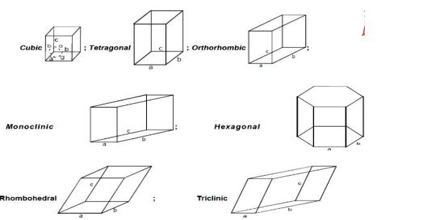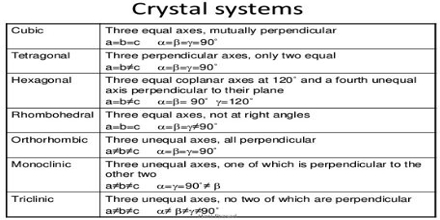Chemistry

# Unit CellUnit cell

The nominal division of a crystal is called a unit cell. It is shaped by the amalgamation of atoms and molecules. The whole crystal structure can be formed by the repetition of these unit cells. The repeating units or cells in the crystal lattice are called unit cells (figure). Each unit cell is defined in terms of lattice points–the points in space about which the particles are free to vibrate in a crystal. The lengths of the edges of a unit cell and the angles between them are called the lattice parameters. The unit cell will have the same symmetry as the crystal. If each point in a space lattice is replaced by an identical atom, ion or group of atoms a crystal structure is obtained. The unit cells can have seven possible shapes (Figure).There are seven crystal systems that atoms can pack together to produce 3D space lattice. Each unit cell has characteristic parameters. A particular crystal may be formed from one of these unit cells and the seven different crystal structures thus formed are called crystal systems.

The characteristics of the crystal system are summarized in Table.Fig: the crystal systems